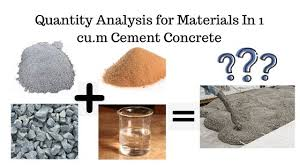### HOW TO CALCULATE REQUIRED QUANTITY OF RAW MATERIAL IN 1CU.M M25 CONCRETE

We all know that the estimation of quantity is very important when we are worked at site. We all should know the method to calculate the quantity of cement, sand and aggregate for purchasing them before to start any concreting work. So below I'll tell you whole method of how to calculate required quantity for 1 cum concrete work.We have M 25 grade of concrete, which proportion is 1:1:2.

Where, cement = 1
Sand = 1
Aggregate = 2.

We need 1 cu.m of wet volume
first of all we need to calculate dry volume of 1 cu.m wet concrete.

Also read How to calculate dry volume of concrete and what is  1.54?

Dry volume = 1.54 X Wet volume
= 1.54 X 1 Cu.m
= 1.54 Cu.m

Now we have to calculate quantity of all ingredients step by step.

For M 25 (1:1:2)

Cement = Dry volume X proportion of cement

Total of all proportion ingredient

= 1.54 X 1
4
= 0.385 cu.m
We know that the 1 bag of cement has 0.0347 cu.m of volume.
Hence,
= 0.385
0.0347
= 11.095 bag
So we have required 11.1 bag of cement.

Sand = 1.54 X 1
4
= 0.385 cu.m
So we have required 0.385 cu.m of sand.

Aggregate = 1.54 X 2
4
= 0.77 cu.m
So we have required 0.77 cu.m of aggregate.